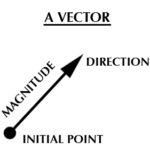# Vector Calculus: DefinitionVector calculus takes the principles of the “one dimensional calculus” of lines and curves and applies them to three-dimensional (or higher) curves, surfaces and solid bodies. A “vector” is a triple of numbers, defined in terms of Cartesian components with respect to a fixed choice of coordinates. Or, from a physicists point of view, it is simply an arrow in space (Dray & Manogue, 1998).

## Vector Calculus vs. Single/Multivariate Calculus

Vector Calculus is concerned with vector-valued functions such as v(t) = − sin(t). By comparison:

The terms multivariable calculus and vector calculus are sometimes used interchangeably, but this isn’t technically correct. Multivariate calculus covers all calculus to do with multivariate functions, which may or may not be vector-valued. Vector calculus could more correctly be described as a sub-field of multivariate calculus.

In the “usual” calculus, integration is performed with ordinary integrals for lines and curves, double integrals for areas, and triple integrals for volume. In vector calculus, these fundamental operations are extended as gradient, curl and divergence.

## Fundamental Theorems of Vector Calculus

Vector calculus has four main theorems, all of which are basically reworkings of the Fundamental Theorem of Calculus to apply to more complex surfaces describe by multivariate functions.

• Gradient theorem: Works with multidimensional curves; relates an integral over the interior of a region to an integral over the region’s boundary.
• Green’s Theorem: The fundamental theorem applied to planes.
• Stokes’ Theorem: Relates the derivative and the boundary to surfaces in three dimensions.
• Divergence Theorem: Extends the theorem to apply to three-dimensional regions bounded by a closed surface.

## References

Breen, J. The Theorems of…. Retrieved September 19, 2020 from: https://www.math.ucla.edu/~josephbreen/The_Theorems_of_Vector_Calculus.pdf
Dray, T. & Manogue, C. (1998). The Vector Calculus Gap: Mathematics ≠ Physics. Retrieved September 19, 2020 from: https://sites.science.oregonstate.edu/math/bridge/papers/calculus.pdf
Oliver, P. Vector Calc in Three Dimensions. Retrieved September 19, 2020 from: https://www-users.math.umn.edu/~olver/ln_/vc3.pdf

CITE THIS AS:
Stephanie Glen. "Vector Calculus: Definition" From StatisticsHowTo.com: Elementary Statistics for the rest of us! https://www.statisticshowto.com/vector-calculus-definition/